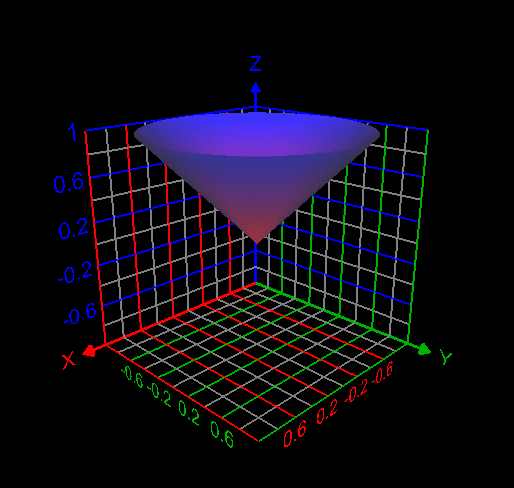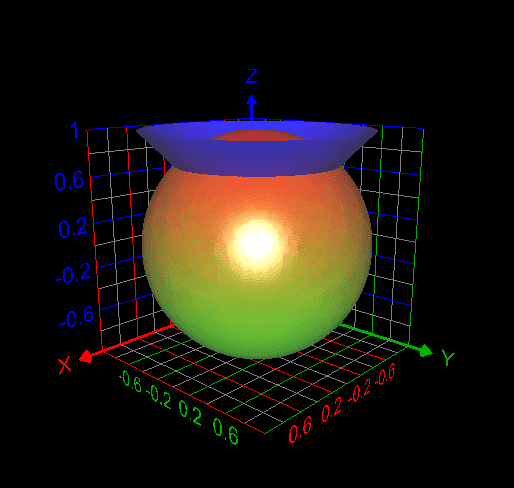# How to use change of variables technique here?

• MHB
• WMDhamnekar

#### WMDhamnekar

MHB
Find the volume V inside both the sphere $x^2 + y^2 + z^2 =1$ and cone $z = \sqrt{x^2 + y^2}$

My attempt: I graphed the cone inside the sphere as follows. But I don't understand how to use the change of variables technique here to find the required volume. My answer without using integrals is volume of the cone + volume of the spherical cap = $\frac{\pi}{3} \times (\frac12) \times \frac{1}{\sqrt{2}} + \frac{ \pi}{3} *(1-\frac{1}{\sqrt{2}})^2 *(\frac{3}{\sqrt{2}}-(1-\frac{1}{\sqrt{2}}))= 0.497286611528$Last edited:

I would change to "cylindrical coordinates" in which z is kept and x and y are replaced by polar coordinates r and $\theta$. The boundary equations become $x^2+ y^2+ z^2= r^2+ z^2= 1$ and $z= r$. The "differential of volume" is $r drd\theta dz$. r goes from 0 to 1, $\theta$ goes from 0 to $2\pi$, and z goes from 0 to 1. The volume is given by $\int_{z= 0}^1\int_{\theta= 0}^{2\pi}\int_{r= 0}^1 rdrd\theta dz$
I would change to "cylindrical coordinates" in which z is kept and x and y are replaced by polar coordinates r and $\theta$. The boundary equations become $x^2+ y^2+ z^2= r^2+ z^2= 1$ and $z= r$. The "differential of volume" is $r drd\theta dz$. r goes from 0 to 1, $\theta$ goes from 0 to $2\pi$, and z goes from 0 to 1. The volume is given by $\int_{z= 0}^1\int_{\theta= 0}^{2\pi}\int_{r= 0}^1 rdrd\theta dz$